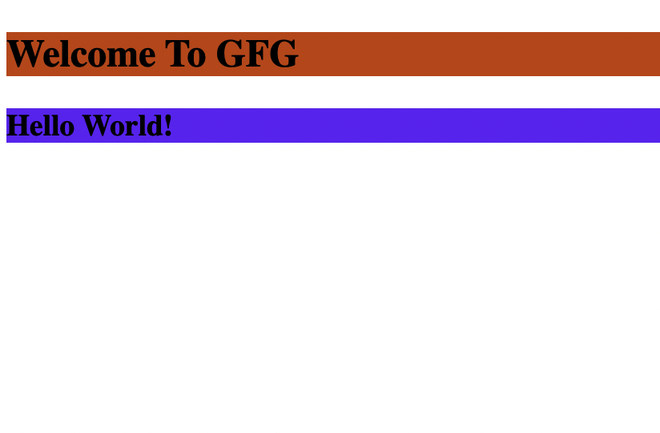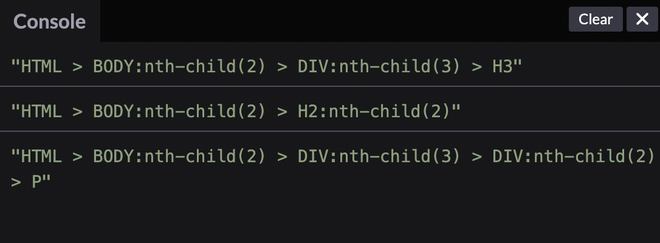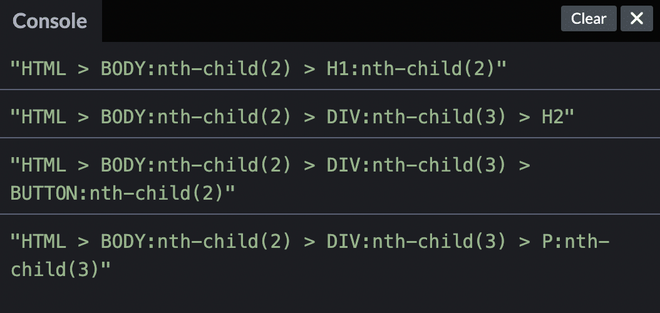Open in App
Not now

# How to create a function `generateSelector` to generate CSS selector path of a DOM element ?

• Last Updated : 24 Aug, 2022

In this article, we will learn about the CSS selectors, and we will also implement a `generateSelector()` function that returns a string value to provide a valid selector to a target DOM element.

What is a CSS Selector?

A CSS selector is used to select a group of elements (or nodes in the DOM tree) that follows a certain pattern. For example –

## HTML

 ```<``html``>`` ` `<``head``>``    ``<``title``>Page Title`` ` `    ``<``style``>`` ` `        ``/* h2 selector selects all the h2 ``        ``elements present in body */``        ``h2 {``            ``background-color: red;``        ``}`` ` `        ``/* div > h3 selector selects all the ``        ``h3 elements which are direct ``        ``descendants of div */``        ``div > h3 {``            ``background-color: blue;``        ``}``    `````` ` `<``body``>``    ``<``h2``>Welcome To GFG``    ``<``div``>``        ``<``h3``>Hello World!``    `````` ` ``

Output –Code output

Understanding the outline concept behind generateSelector() Function: Now, let’s talk about how to create a `generateSelector()` function which returns a valid selector string to a target DOM element that presents inside a DOM tree. For a better understanding, let’s take a look at some of the examples of the function and its expected response.

Example:

## HTML

 ```<``html``>`` ` `<``head``>``    ``<``title``>Page Title```` ` `<``body``>``    ``<``h2``>Welcome To GFG``    ``<``div``>``        ``<``h3``>Hello World!``        ``<``div``>``            ``<``p``>Some random text``        ````    ```` ` `    ``<``script``>``        ``let target = document.querySelector('h3');``        ``generateSelector(target);`` ` `        ``// Expected output - ``        ``//"HTML > BODY:nth-child(2) > DIV:nth-child(3) > H3"`` ` `        ``target = document.querySelector('h2');``        ``generateSelector(target);``        ``// "HTML > BODY:nth-child(2) > H2:nth-child(2)"`` ` `        ``target = document.querySelector('p');``        ``generateSelector(target);``      ``// "HTML > BODY:nth-child(2) > DIV:nth-child(3) ``      ``// > DIV:nth-child(2) > P"``    `````` ` ``

The above code is just a pseudo code that does not have the implementation of generateSelector function. It is only there to help you understand what the function is for. We are going to implement this function down below in the next section –

Expected Output:

HTML > BODY:nth-child(2) > DIV:nth-child(3) > H3
HTML > BODY:nth-child(2) > H2:nth-child(2)
HTML > BODY:nth-child(2) > DIV:nth-child(3) > DIV:nth-child(2) > P

Implementing `generateSelector()` function: Now, we have a good grasp on what `generateSelector` function is, let’s try to implement it –

## HTML

 ```<``html``>`` ` `<``head``>``    ``<``title``>Page Title```` ` `<``body``>``    ``<``h2``>Welcome To GFG``    ``<``div``>``        ``<``h3``>Hello World!``        ``<``div``>``            ``<``p``>Some random text``        ````    ```` ` `    ``<``script``>``        ``// Let's write a generateSelector() function``        ``// that should return selector path to h3``        ``// inside the div``        ``const generateSelector = (target) => {``            ``const selectorPath = [];`` ` `            ``while (target.tagName) {``                ``let i = 0;`` ` `                ``if (target.parentNode) {``                    ``const children = target.parentNode.children;`` ` `                    ``while (i < ``children.length` `&& children[i] !== target) {``                        ``i++;``                    ``}``                ``}`` ` `                ``selectorPath.unshift(target.nodeName + (``                    ``i > 0 ? `:nth-child(\${i + 1})` : ''));``                ``target = target.parentNode;``            ``}`` ` `            ``return selectorPath.join(' > ');``        ``}`` ` `        ``let target = document.querySelector('h3');``        ``console.log(generateSelector(target));``        ``// Expected output - ``        ``// "HTML > BODY:nth-child(2) > DIV:nth-child(3) > H3"`` ` `        ``target = document.querySelector('h2');``        ``console.log(generateSelector(target));``        ``// "HTML > BODY:nth-child(2) > H2:nth-child(2)"`` ` `        ``target = document.querySelector('p');``        ``console.log(generateSelector(target));``      ``// "HTML > BODY:nth-child(2) > DIV:nth-child(3) ``      ``// > DIV:nth-child(2) > P"``    `````` ` ``

Let’s break the above code step by step –

• We create a selectorPath array to store selector elements in reverse order (as we bubble up the DOM tree from the target element)
• We run a loop to travel up the DOM tree until we reach a NULL, i.e. until the root of the DOM tree is traversed.
• We run through the children of the target’s parentNode until we reach the target itself. This is done so as to determine the index of the target among its parent’s children so that we can add it to the selector.
• We update the selectorPath array with the current selector path value and also update the target to its parentNode.
• We continue doing the same until the whole DOM tree is traversed.
• After the loop is over, we join the selectorPath array elements together with ‘ > ‘ as a concatenate symbol, which signifies a child of a parent is a CSS selector.
• The resultant string is the final CSS selector string that we were looking for.

Output:Code output

Example 2 –

## HTML

 ```<``html``>`` ` `<``head``>``    ``<``title``>Page Title```` ` `<``body``>``    ``<``h1``>Welcome to GFG``    ``<``div``>``        ``<``h2``>Random text``        ``<``button``>Click me``        ``<``p``>Hello world``    ```` ` `    ``<``script``>``        ``// Let's write a generateSelector() function``        ``// that should return selector path to h3``        ``// inside the div``        ``const generateSelector = (target) => {``            ``const selectorPath = [];`` ` `            ``while (target.tagName) {``                ``let i = 0;`` ` `                ``if (target.parentNode) {``                    ``const children = target.parentNode.children;`` ` `                    ``while (i < ``children.length` `&& children[i] !== target) {``                        ``i++;``                    ``}``                ``}`` ` `                ``selectorPath.unshift(target.nodeName + ``                    ``(i > 0 ? `:nth-child(\${i + 1})` : ''));``                ``target = target.parentNode;``            ``}`` ` `            ``return selectorPath.join(' > ');``        ``}`` ` `        ``let target = document.querySelector('h1');``        ``console.log(generateSelector(target));`` ` `        ``target = document.querySelector('h2');``        ``console.log(generateSelector(target));`` ` `        ``target = document.querySelector('button');``        ``console.log(generateSelector(target));`` ` `        ``target = document.querySelector('p');``        ``console.log(generateSelector(target)); ``    `````` ` ``

The above example is implemented in the same way as Example 1, but it has different child elements in the body tag, and so the different outputs get logged to the console.

Output:My Personal Notes arrow_drop_up